# VBA VLOOKUP & XLOOKUP

This article will demonstrate how to use the VLOOKUP and XLOOKUP functions in VBA.

The VLOOKUP and XLOOKUP functions in Excel are extremely useful. They can also be used in VBA Coding.

## VLOOKUP

There are two ways to call the VLOOKUP Function in VBA:

• Application.WorksheetFunction.Vlookup
• Application.Vlookup

The two methods worked identically, except for how they handle errors.

### Application.WorksheetFunction.VLookup

This example will demonstrate the first method.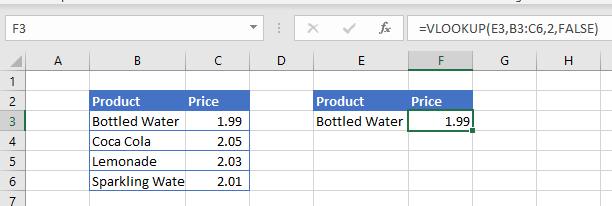Here we’ve created a VLOOKUP formula in Excel that we will replicate in VBA:

``````Sub LookupPrice()
ActiveCell = Application.WorksheetFunction.VLookup(Range("E3"), Range("B2:C6"), 2, False)
End Sub``````

This will result in the following: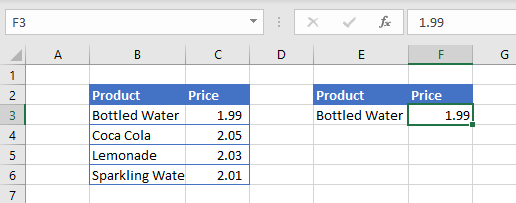Note that the actual result was written to cell F3 instead of the formula!

If we wish to return a formula to Excel instead of a value, write the VLOOKUP into a formula:

``````Sub LookupPrice()
ActiveCell = "=VLOOKUP(E3,B2:C6,2,FALSE)"
End Sub``````

Or you can write the formula to a cell using R1C1 notation, which creates a formula with relative references that can be used over a range of cells:

``````Sub LookupPrice()
ActiveCell = "=VLOOKUP(RC[-1],RC[-4]:RC[-3],2,FALSE)"
End Sub``````

You can also use variables to define the VLOOKUP inputs:

``````Sub LookupPrice()
Dim strProduct As String
Dim rng As Range
strProduct = Range("E3")
Set rng = Range("B2:C6")
ActiveCell = Application.WorksheetFunction.VLookup(strProduct, rng, 2, False)
End Sub``````

Of course, instead of writing the results of the function to a cell, you can write the results to a variable:

``````Sub LookupPrice()
Dim strProduct As String
Dim rng As Range
Dim strResult as String

strProduct = Range("E3")
Set rng = Range("B2:C6")
strResult = Application.WorksheetFunction.VLookup(strProduct, rng, 2, False)
End Sub``````

### Application.VLookup vs Application.WokrsheetFunction.VLookup

In the examples above, we can use either of the methods and they will return the same result. However what if we were looking up a Product that doesn’t exist – what result would this return?  Each function would return a very different result.

Normally when using VLOOKUP in Excel, if the Lookup does not find the correct answer, it returns #N/A back to the cell. However, if we use the example above and look for a product that does not exist, we will end up with a VBA Error.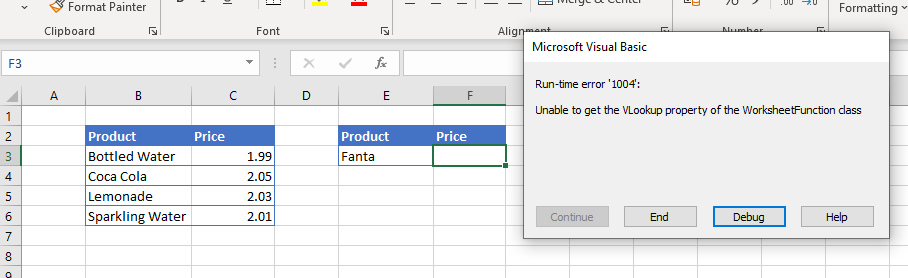We can trap for this error in our code and return a more user friendly message when the item we are looking for is not found.

``````Sub LookupPrice()
On Error GoTo eh
ActiveCell = Application.WorksheetFunction.VLookup(Range("E3"), Range("B2:C6"), 2, False)
Exit Sub
eh:
End Sub``````

Alternatively, we can amend our VBA code to use Application.Vlookup instead of Application.WorksheetFunction.VLookup.

``````Sub LookupPrice()
ActiveCell = Application.VLookup(Range("E3"), Range("B2:C6"), 2, False)
End Sub``````

When we do this, the #N/A that we have come to expect when a lookup value is not found, is returned to the cell.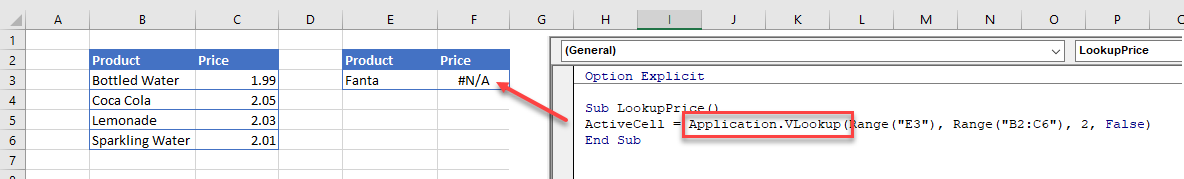## WorksheetFunction.XLOOKUP

The XLOOKUP function has been designed to replace the VLOOKUP and HLOOKUP functions in Excel. It is only available to Office 365 so using this function is quite restrictive is some of your users are using older versions of Excel.

It works in much the same ways in VBA as the VLOOKUP function.

``````Sub LookupPriceV()
ActiveCell = Application.WorksheetFunction.XLookup(Range("E3"), Range("B2:B6"), Range("C2:C6"))
End Sub``````

OR

``````Sub LookupPriceV()
ActiveCell = Application.XLookup(Range("E3"), Range("B2:B6"), Range("C2:C6"))
End Sub``````

Once again, if we wish to use variables, we can write the following code

``````Sub LookupPriceV()
Dim strProduct As String
Dim rngProduct As Range
Dim rngPrice As Range
strProduct = Range("E3")
Set rngProduct = Range("B2:B6")
Set rngPrice = Range("C2:C6")
ActiveCell = Application.WorksheetFunction.XLookup(strProduct, rngProduct, rngPrice)
End Sub``````

If we wish to return a formula to Excel instead of a value, we would need to write the formula into Excel with the VBA code.

``````Sub LookupPrice()
ActiveCell = "=XLOOKUP(E3,B3:B6,C3:C6)"
End Sub``````

Or you can write the formula to a cell using R1C1 notation.

``````Sub LookupPrice()
ActiveCell.Formula2R1C1 = "=XLOOKUP(RC[-1],RC[-4]:RC[-4],RC[-3]:RC[-3])"
End Sub``````

One of the major advantages of using XLOOKUP vs VLOOKUP is the ability to lookup a range of columns, and return the value in each column.

Lets look at the following example: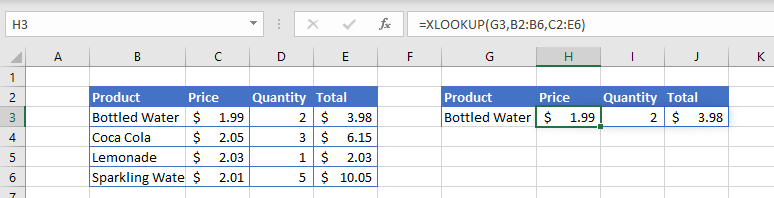We have created the formula in cell H3 where it is looking up the values in the range C2:E6.  Due to the fact that these are multiple columns, it will automatically populate columns I and J with the matching results found.  The formula SPILLS over into columns I and J without us having to use CTRL+SHIFT for an array formula – this ability for the formula to SPILL across is one of the new additions for Excel 365.

To replicate this with VBA code, we can type the following in our macro:

``````Sub LookupPrice()
ActiveCell.Formula2R1C1 = "=XLOOKUP(RC[-1],RC[-6]:RC[-6],RC[-5]:RC[-3])"
End Sub``````

Where the formula is using the R1C1 (rows and columns) syntax instead of the A1 (range) syntax.   This will result in the formulas being entered into Excel as shown in the graphic above.

You cannot lookup multiple columns if you are using the WorksheetFunction method.